• mat.type()函数opencv中Mat存在各种类型，其中mat有一个type()的函数可以...具体的有以下值：通道数我们可以发现，C4=C3+8、C3=C2+8 c++ opencv mat 最大值#include using namespace cv;using namespace std;int ma...

mat.type()函数

opencv中Mat存在各种类型，其中mat有一个type()的函数可以返回该Mat的类型。类型表示了矩阵中元素的类型以及矩阵的通道个数，它是一系列的预定义的常量。具体的有以下值：

通道数我们可以发现，C4=C3+8、C3=C2+8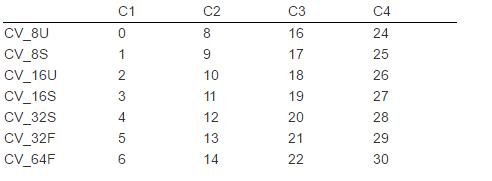c++ opencv mat 最大值

#include

using namespace cv;

using namespace std;

int main()

{

uchar data[] = {

10, 200, 5, 7, 9,

15, 35, 60, 80, 170,

100, 2, 55, 37, 70

};

Mat m1(3, 5, CV_8U, data);

Mat m_min, m_max;// minimum value, maximum value

double minVal, maxVal;

int minIdx = {}, maxIdx = {};// minnimum Index, maximum Index

minMaxIdx(m1, &minVal, &maxVal, minIdx, maxIdx);

cout << "[m1] = " << endl << m1 << endl << endl;

cout << "[m_min] = " << endl << m_min << endl;

cout << "[m_max] = " << endl << m_max << endl << endl;

cout << "minimum value in m1 : " << minVal << endl;

cout << " minimum value's Index is : " << minIdx << ", " << minIdx << endl;

cout << "maximum value in m1: " << maxVal << endl;

cout << " maxnimum value's Index is : " << maxIdx << ", " << maxIdx << endl << endl;

return 0;

}

展开全文• C语言：用指针求最大值和最小值 用指针数组最大值和最小值(10分) 题目内容: 用指针含有十个元素的数组最大值和最小值 主函数参考 int main() { int a,i,maxnum,minnum; for(i=0; ... python 版 mldivide ...

函数格式

scipy.optimize.linprog(c, A_ub=None, b_ub=None, A_eq=None, b_eq=None, bounds=None, method='simplex', callback=None, options=None)

今天阅读数据建模第一章线性规划问题，问题描述如下：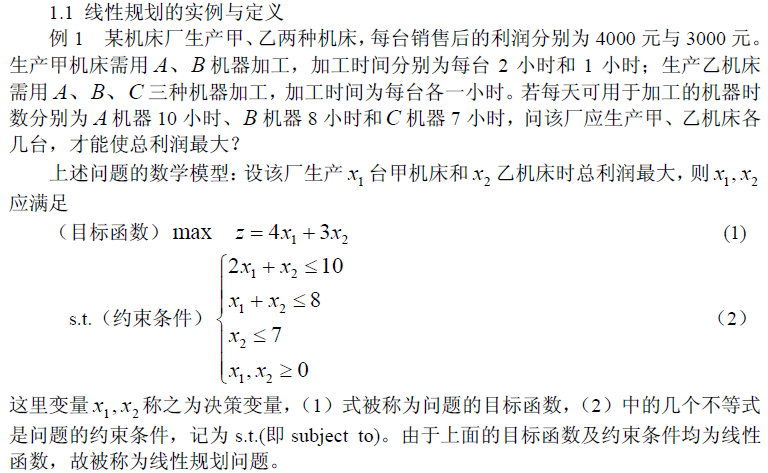通过介绍我们知道了线性规划，就是目标函数及约束条件均为线性函数。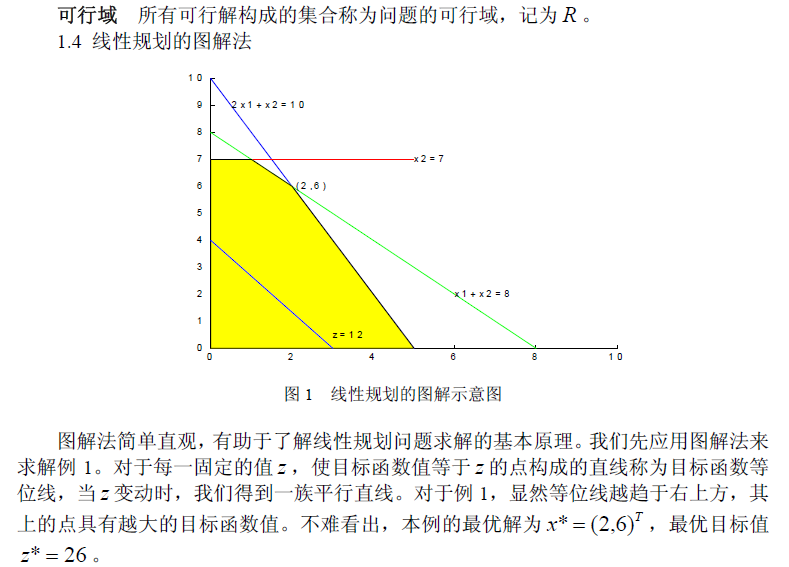通过画图我们可知，X1，X2的最优解为2，6，目标值为26。

我们如何时候这个scipy的公式来计算这个值呢:

>>> c = [-1, 4]

>>> A = [[-3, 1], [1, 2]]

>>> b = [6, 4]

>>> x0_bounds = (None, None)

>>> x1_bounds = (-3, None)

>>> from scipy.optimize import linprog

>>> res = linprog(c, A_ub=A, b_ub=b, bounds=(x0_bounds, x1_bounds),

... options={"disp": True})

>>> print(res)

Optimization terminated successfully.

Current function value: -11.428571

Iterations: 2

status: 0

success: True

fun: -11.428571428571429

x: array([-1.14285714, 2.57142857])

message: 'Optimization terminated successfully.'

nit: 2

上面是官方给出的案例，我们很难看出来这个怎么求解最大值，不过英语好的也可以把。

言归正传，我们先结合官网是思路得出最小值的解。

In :c = np.array([4,3])

In :a = np.array([[2,1],[1,1]])

In :In :b = np.array([10,8])

In :optimize.linprog(c,a,b,bounds=((0,None),(0,7)))

Out:

fun: -0.0

message: 'Optimization terminated successfully.'

nit: 0

slack: array([ 10.,   8.,   7.])

status: 0

success: True

x: array([ 0.,  0.])

按照正常的计算 我们得出了最小值为0，且x的两个值为 0 ， 0。对于上面的的公式有必要说明的是，bounds是针对x的最大最小一次给一个值，从题目可知，x1的取值范围为大于0，最小值就为0，最大值没有约束，被其他的条件所约束就可以了 ，没有明确，所以是写的 (0，None),而相对x2来说，他最小值为0，最大值被C约束，为7。所以范围为(0，7)，当有三个求解的时候，依次增加，不可省略。

接下来我们说这个最大值怎么求，其实只要对C取反我们就可以求除最大值的负数，对结果在取反回来就可以了

In :optimize.linprog(-c,a,b,bounds=((0,None),(0,7)))

Out:

fun: -26.0

message: 'Optimization terminated successfully.'

nit: 2

slack: array([ 0., 0., 1.])

status: 0

success: True

x: array([ 2., 6.])

是不是很简单，得到的-26取反回来就是我们的最大值求解了，(2，6)就是我们的X1,X2取值了。其实有时候那个条件为>,>=的时候我们要写成

我这里在补充一个求三个解的实例：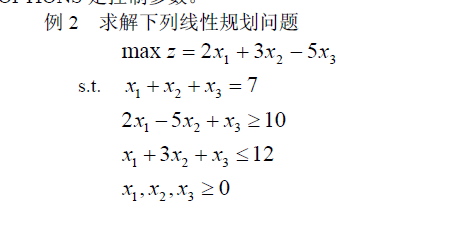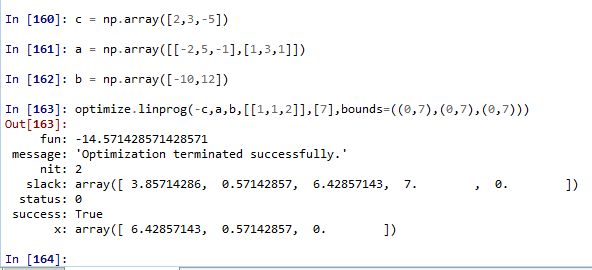我们的最优解为 14.57

使用python scipy&period;optimize linprog和lingo线性规划求解最大值，最小值(运筹学学习笔记)

1.线性规划模型: 2.使用python scipy.optimize linprog求解模型最优解: 在这里我们用到scipy中的linprog进行求解,linprog的用法见https://doc ...

POJ 3264 Balanced Lineup【线段树区间查询求最大值和最小值】

Balanced Lineup Time Limit: 5000MS   Memory Limit: 65536K Total Submissions: 53703   Accepted: 25237 ...

【BigData】Java基础&lowbar;定义工具类，对ArrayList排序并且求最大值、最小值、平均值

需求描述 编写一个工具类,对ArrayList实现以下功能: ① 排序 ② 求最大值 ③ 求最小值 ④ 求平均值 需求实现 实现代码 package cn.test.logan.day04; impo ...

C语言：用指针求最大值和最小值

用指针求数组最大值和最小值(10分) 题目内容: 用指针求含有十个元素的数组最大值和最小值 主函数参考 int main() { int a,i,maxnum,minnum; for(i=0; ...

python 版 mldivide matlab 反除(左除)《数学建模算法与程序》Python笔记

今天在阅读数学建模的时候看到了差分那章 其中有一个用matlab求线性的代码,这里我贴出来 这里我送上 Python代码 In : import numpy as np ...: from s ...

JS数组求最大值和最小值

展开全文c语言线性规划求最值
• 本次实验的目的是得到每个组合中达最优值（最小值 456.7）的迭代次数的最小值、最大值、均值以及方差。 data.txt 代码实现 1. matlab读取文件，并输出“The minimal is 456.7” %% 读取数据 fid = fopen('matlab...

最近做了一个matlab实验任务，主要是将数据文档中的数据进行处理。考虑到数据文件的保密性，本文就简单介绍一下实验中用到的函数和语法。

实验数据以及实验目的

新建了一个数据文件data.txt，文件内容主要包括最小value(第一行)；方案组合(color，size，length)；表头(run no., number, value);以及具体的数字。

-run no.——运行次数序号，每个组合运行五次；

-number——每次运行中第一次达到最优值的迭代次数；

-value——运行得到的最优值；

本次实验的目的是得到每个组合中达最优值(最小值 456.7)的迭代次数的最小值、最大值、均值以及方差。data.txt

代码实现

1. matlab读取文件，并输出“The minimal is 456.7”

%% 读取数据

fid = fopen('matlab实验-简书\data.txt','r+');

FormatString=repmat('%s',1,3);

A1=A{1};

A2=cell2mat(A1);

formatSpec ='The minimal is %s.\n';

fprintf(formatSpec,A2)

2. 输出列名

%% 输出列名

s1=' ';

num=' num';

m1=' min';

m2=' max';

m3=' mean';

fdev=' fdev';

fprintf('%s %s %s %s %s %s\n',s1,num,m1,m2,m3,fdev);

3. 处理行名及数据内容

%% 处理行名及求数据

for x=1:7:14

%处理行名，将组合名称缩写

fid = fopen('matlab实验-简书\data.txt','r+');

B1=B{1};

B2=cell2mat(B1);

line_short=strrep(B2,'red','re');

line_short=strrep(line_short,'blue','bl');

line_short=strrep(line_short,'big','bi');

line_short=strrep(line_short,'small','sm');

line_short=strrep(line_short,'long','lo');

line_short=strrep(line_short,'short','sh');

%

C1=C{3};

C1C=C{2};

C2=cell2mat(C1);

%将每个组合运行5次中达到最小值的number放进矩阵

C3=string(C2);

C4=vpa(C3,6);

C5=string(A2);

i=1;

xx=strings;

for y=1:5

if (C3(y)==C5)

yy=C1C{y};

xx(i)=yy;

i=i+1;

end

end

C6=double(xx);

le=length(C6(:));

count=le;

%计算每个组合5次运行中达到最小值的number的最小值、最大值、均值

data_min=min(C6(:));

data_max=max(C6(:));

C9=mean(C6(:));

data_mean=vpa(C9,4);

C11=0;

%计算方差

for j=1:le

C11=C11+(C6(j)-C9)^2;

end

C12=C11./(le-1);

data_fdev=vpa(C12,6);

%打印数据

fprintf('%s:%6.0f %6.0f %6.0f %7.0f %13.3f\n',line_short,count,data_min,data_max,data_mean,data_fdev);

fclose(fid);

end

代码结果

最终的实验结果如下：实验结果

注：

本次实验代码不严谨，只是保证结果运行策略，没有进行规范化书写，毕竟只是个作业哈~

展开全文• %分解成4*4B=mat2cell(A,ones(N/M,1)*M,ones(N/Mmatlab中矩阵之间赋值=a(:)得到的b是一个列向量.b=a得到的是一个行向量.诚教:matlab中取矩阵的其中几行几列如果想取一个矩阵其中的第1,3,5,6,8行,为一...

Matlab中矩阵分块问题

下面给你弄一个小的矩阵分块的示范.你的256*256类似地改一下：N=16;%16*16A=magic(N)M=4;%分解成4*4B=mat2cell(A,ones(N/M,1)*M,ones(N/M

matlab中矩阵之间赋值

=a(:)得到的b是一个列向量.b=a得到的是一个行向量.

诚教:matlab中取矩阵的其中几行几列

如果想取一个矩阵其中的第1,3,5,6,8行,为一个新的矩阵,则用下列语句：B=A([1,3,5,6,8],:);如果是取第1,3,5行和第1,4,5列相交的数据为新矩阵,可以表示为：C=A([1,3

请问 matlab中矩阵 A.'

matlab默认的矩阵转置是共轭转置,是对复数进行操作的.求共轭转置矩阵的指令为A'非共轭转置矩阵的指令为A.',相当于conj(A')由于一般输入的矩阵都是实矩阵,所以A'后就是conj(A'),也

请问如何在matlab中,删除二维矩阵每行的一个0元素?

这个问题确实很有意思,我好歹弄出来了,不过还是用了for循环,请参阅,看看能不能修改clear;clc;closealla=[1023;0021;1103];[m,n]=size(a);[b,c]=f

matlab中矩阵的建立

A=zeros(1,24);B=ones(1,25);A=B(2:25)如此即可,冒号的意思是从第2个元素到第25个.

matlab 矩阵中元素分割

设源矩阵叫MAT：A=floor(MAT/1e6);B=mod(MAT,1e6);再问：请问如果想保留4-11位该怎么操作呢？再答：级联操作就好了：C=floor(mod(MAT,1e11)/1e3)

matlab中创建以下矩阵?

A＝［1：4：12］；A(6)＝12　；B＝[2436;1532;2779;1235];C＝B＾3；D1＝［B,C］；D＝D1(2：4,2：7)；E＝［B；C］；F＝E(3：5,2：3)；G＝resh

matlab中怎样取一个矩阵的连续很多列

你的行没有输入,应该是P_test=P[,1:60].如果只写1:60,matlab会认为是按位置从第一个元素开始先按列提取,再按行提取.找本matlab的书看吧,一般前几章就会有这部分内容.

matlab中输入矩阵问题

x(1:10)=1:10%元素值等于下标值i=1:10x(i)=2*i+1%线性关系%其他的发挥你的想象力

matlab中矩阵合并

用元胞数组,cell再问：高人能不能给个具体的用法？

matlab中矩阵套矩阵问题

这么写确实挺奇怪的,感觉就是根据C中的坐标提取A,B中的对应数据.其实,写三个小点的矩阵试一下就就知道了再问：Thankyuo,已经试出来了，谢谢

Matlab怎样从数组矩阵中随机取数?

tryfunction'randperm',forexample:a=;b=a(randperm(length(a)));x=b(1:5);

MATLAB中矩阵每行都是一样共100行

fori=1:100;A1=;A(i,:)=A1;endA

求助利用Matlab编程：矩阵中每行各取一个元素,使其和最小,并给出该最短路径的坐标

分析：矩阵中每行各取一个元素,使其和最小,那么如果每行都取的是该行的最小值的话,那么最后的和肯定也是最小的.所以只需找到每行的最小值即可.%%%%%%%%%%%%%%%%%%%%%%%%%%%%%%%

怎么样让matlab中矩阵每行的列数不一样长

细胞矩阵,每个元素中的内容可以任意大小你这种定义一个NX1的细胞矩阵即可细胞矩阵的具体定义可以百度或者定义一个类(matlab也支持面向对象)然后定义一个关于类的矩阵,具体如A(1).name=‘zh

matlab如何计算矩阵的每行元素中每三列平方和(一行中每三列计算一次)

ct=5;A=randint(3,3*ct,)%A用自己的数据[m,n]=size(A);B=reshape(A,m*ct,3);R=sum(B.^2,2);R=reshape(R,m,ct

MATLAB中矩阵相加

输入时不是在英文状态下,你可以看到标点是中文的,换输入状态试试.或者你输入错了,x(:,2))输成了x1(:,2))再问：不是这个问题，你用自己电脑的matlab复制试试。我要画的是X=X1+X2，不

展开全文• 写项目的时候遇到了 过两天把他代码学东优化好更新一下 （一）目的 ...实现1个矩阵（256*1024）最大值计算，初始值随机产生，值的范围限定在[1-2018]。 实现4个版本，分别计算运行时间 版本1：C...cuda
• console.log(max)由于max()里面参数不能为数组，所以借助apply(funtion,args)方法调用Math.max()，function为要调用的方法，args是数组对象，当function为null时，默认为上文,即相当于apply(Mat...
• 在MATLAB中从3个矩阵中获取坐标最大值(Get coordinate-wise maximum out of 3 matrices in MATLAB)假设我有3个矩阵A ， B ， C 。 我想创建一个包含矩阵最大值的新矩阵。例如，让A = [ a11 a12.. ] B = [b11 b12 ..]...
• 我想通过搜索所有图像找到每个像素位置的最大值和索引.以下是示例表示： 我的代码：imgs = cell(1,5);imgs{1} = [2,3,2;3,2,2;3,1,1];imgs{2} = [2,3,1;4,2,3;2,2,1];imgs{3} = [3,2,1;5,3,5;3,2,3];imgs{4} = [4,4,...
• 2、递归局部问题 3、将局部问题的解"整合"，解决原问题 //分治法找最大元素模板题（最小） #include <bits/stdc++.h> using namespace std; /*大白2书上p110有问题： int fmax(int a[],int l,int r){ ...
• Maybe I'm not looking hard enough, but everything seems to want me to use an ... Thus, how do I get the channel value for a particular pixel for foo if foo is something like Mat foo = imread("bar.p...
• 采用统计学方式进行kernal计算不仅有中值滤波，还有最大值与最小值滤波。顾名思义，最值滤波就是取kernal排序后得最大值或最小值来取代中心像素作为输出。不过在OpenCV中并无max或min滤波函数，而是以形态学操作...opencv 人工智能 计算机视觉 javafx
• 这次给大家带来怎样在数组中取最大值，在数组中取最大值的注意事项有哪些，下面就是实战案例，一起来看一下。1.自己喜欢的方式；let arr = [1,2,3,4,5,5,6,7];console.log(1,Math.max.apply(Math,arr));let arr2 = ...php数组取最大值
• OpenCV Mat 介绍

2021-01-13 18:06:18
1. 为什么要引入 Mat 图像容器？在计算机看来，一幅图像对应的是矩阵，矩阵包含了所有像素点的强度。获取并存储这些像素，可以使计算机图像处理简化为数值矩阵及描述矩阵信息的处理。OpenCV 是如何存储图像的呢...c++ mat赋值
• 突然发现有一篇opencv操作mat数据的基础操作搞忘了，现在发出来，原来有一篇是只有操作结果,而且是用emgu写的，这里用c++实现并给出详细的代码。数字图像处理其实就是处理二维矩阵数据。利用opencv来学习处理算法是...c++ mat赋值
• #include <opencv2/opencv.hpp> #include <iostream> #include <...void MinFilter(Mat &src_image, Mat &dst_image, int k_size = 3) { int max_rows = src_image.rows; //c++
• OpenCV Mat 类型定义和赋值

千次阅读 2020-12-24 10:47:26
1.一般的Mat定义方法：cv::Mat M(height,width,)，例：cv::Mat M(480,640,CV_8UC3); 表示定义了一个480行640列的矩阵，矩阵的每个单元的由三个(C3:3 Channel)8位无符号整形(U Unsigned U8 8位)构成。2.将已有数组赋...
• MAT使用

2021-08-09 00:06:29
MAT使用 Eclipse Memory Analyzer 使用 设置 1.运行时堆内存配置 /Applications/mat.app/Contents/Eclipse/MemoryAnalyzer.ini -Xmx1024m 2.选项配置 ![[Pasted image 20210804140711.png]] Keep unreachable ...
• Matlab计算热像图序列的平均和方差1. 文件、数据介绍。2.运行程序，结果如下。3.源代码。 1. 文件、数据介绍。 文件夹下有55个格式为mat的热像图数据。 每个mat文件包含一个200*300的juzhen变量。 2.运行程序，...matlab 图像处理 数学
• 调用这个Math.Random()函数能够返回带正号的double，该大于等于0.0且小于1.0，即取值范围是[0.0,1.0)的左闭右开区间，返回值是一个伪随机选择的数，在该范围内(近似)均匀分布。例子如下：package IO;import java...
• Java内存分析利器MAT使用详解

千次阅读 2021-03-04 10:04:39
这是一篇阅读MAT helper的笔记。Heap dump是java进程在特定时间的一个内存快照。通常在触发heap dump之前会进行一次full gc，这样dump出来的内容就包含的是被gc后的对象。dump文件包含的内容：1，全部的对象：类，域...java mat使用
• 函数求值公式： 如果 order=0 dst=(src-delta)*(src-delta)T 否则 dst=(src-delta)T*(src-delta) [编辑] Trace 返回矩阵的迹 CvScalar cvTrace( const CvArr* mat ); mat 输入矩阵 函数 cvTrace 返回矩阵mat的对角...
• 在MATLAB图像处理工具箱(Image Processing Toolbox)的GUI函数中，有一些用来在图像或坐标轴上选择矩形或椭圆区域...返回矩形区域axes对象的句柄h，MATLAB的help解释为：h = imrect(hparent, position)createsa dr...
• 除以最大值是以最大值为1，得到的是相对的色彩信息。 个人认为，对于亮暗差别不大的，除最大值比较好； 对于亮暗差别比较大的，除255比较好。 目的是提升归一化后的数据对比度。跟调节色彩平稳的软件中的曲线调节...java mat类型
• Mat¶在2001年刚刚出现的时候，OpenCV基于 C 语言接口而建。为了在内存(memory)中存放图像，当时采用名为 IplImage 的C语言结构体，时至今日这仍出现在大多数的旧版教程和教学材料。但这种方法必须接受C语言所有的...
• 目的一个实对称矩阵的所有特征和特征向量。前置知识对于一个实对称矩阵$A$，必存在对角阵$D$和正交阵$U$满足$$D=U^TAU$$$D$的对角线元素为$A$的特征，$U$的列向量为$A$的特征向量。定义$n$阶旋转矩阵G(p,q,\...
• Histogram -> 可以列出内存中的对象，对象的个数以及大小。... 通过图形列出最大的object。Leak Suspects -> 通过MA自动分析泄漏的原因。Objects -> 类的对象的数量，这个对象被创建了多少个Shallow Heap ...
• 需要看一下这些对象能不能被回收以及它为什么没有被回收 with outgoing references：这个类引用了哪些对象 with incoming references：这个类被哪些对象所引用 dominator_tree与Histogram中中这两个的区别是： ...java JVM
• python 矩阵（mat）操作

千次阅读 2021-01-12 23:26:40
a1=mat(a1);data1=mat(zeros((3,3)));#创建一个3*3的零矩阵，矩阵这里zeros函数的参数是一个tuple类型(3,3)data2=mat(ones((2,4)));#创建一个2*4的1矩阵，默认是浮点型的数据，如果需要时int类型，可以使用dtype=int...
• 4、OpenCV中的Mat类

千次阅读 2021-01-06 15:36:28
OpenCV 中的Mat类一、学习目标二、Mat 介绍（建议细读）三、Mat的存储方法四、显示地创建 Mat 对象五、格式化输出 Mat 对象六、其他常见元素的输出七、致谢 一、学习目标 我们有多种方法从现实世界中获取数字图像：...OpenCV教程 OpenCV笔记 OpenCV的Mat Mat对象...